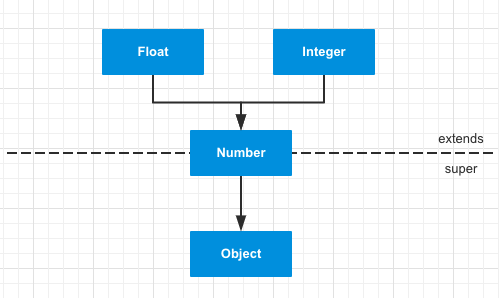# Java&Kotlin在泛型方面的区别

## 泛型¶

Java

// 泛型方法，带泛型返回值
public static <T> T getMiddle(T... a) {
return a[a.length / 2];
}

// 泛型方法
public static <T> void printMiddle(T... a) {
System.out.println("printMiddle = " + a[a.length / 2]);
}

// 泛型类型限定
public static <T extends Comparable<T>> int compare(T t1, T t2) {
return t1.compareTo(t2);
}

// 泛型类型多个限定
public static <T extends Comparable & Serializable> void printArray(T[] input) {
Arrays.sort(input);
System.out.print("sorted: ");
for (T t: input) {
System.out.print(t + "\t");
}
System.out.println();
}

// 泛型类
public static class A<T> {
// 获取泛型T的实际类型
public String getType() {
ParameterizedType parameterizedType = (ParameterizedType) getClass().getGenericSuperclass();
return parameterizedType.getActualTypeArguments().toString();
}

public T getT(T t) {
return t;
}
}


Kotlin

// 泛型方法，带泛型返回值
fun <T> getMiddle(vararg a : T) : T {
return a[a.size / 2]
}

// 泛型方法
fun <T> printMiddle(vararg a : T) {
println("printMiddle = ${a[a.size / 2]}") } // 泛型类型限定 fun <T : Comparable<T>> compare(t1: T, t2: T): Int { return t1.compareTo(t2) } // 泛型类型多个限定，注意多限定时需要使用where关键词 fun <T> printArray(input: Array<T>) where T : Comparable<T>, T : java.io.Serializable { input.sort() print("sorted: ") for (t in input) { print("$t\t")
}
println()
}

// 泛型类
open class A<T> {
// 获取泛型T的实际类型
fun getType(): String = (javaClass.genericSuperclass as ParameterizedType).actualTypeArguments.toString()

fun getT(t: T): T = t
}


Gson().fromJson(it.values, object : TypeToken<List<CatBean>>() {}.type)


println("A.getType() = \${object : A<Int>(){}.getType()}")


A.getType() = class java.lang.Integer


### extends、super通配符¶

extends、super与通配符 ? 搭配可以造成不同的效果。

• List<? extends Number> 表示上限通配符，可以读出Number数据，但是不能添加任何对象
• List<? super Number> 表示下限通配符，可以存入Number及其子类类型的对象，但是取出的时候只能用Object取出
• List<?> 表示无限定通配符，等价于List<? extends Object>Number的部分继承关系

List<? extends Number>中，泛型的具体类型在运行时只能是一个特定的，该类型是Number或者Number的子类都可以，但是由于不确定是哪个具体的类，所以不能添加任何对象，因为添加的对象可能不兼容。比如说，当里面存放的是Float时，我们动态的添加Integer，这是不允许的。同时，从其中读取数据，数据的类型肯定是Number类型的。

List<? super Number>含义与上面相反，泛型的具体类型在运行时只能是一个特定的，该类型是Number或者Number的父类都可以。因此，Number以及其父类都是可以添加进去的。正是因为这个原因，在取出时，所以无法找到一个最高公共父类，除了Object，所以取出时只能是Object类型了。

    public static void getData(List<?> data) {
Integer integer = new Integer(1);
Object object = new Object();
Number number = new Number() {...};

object = data.get(0);
}

public static void getData2(List<? extends Number> data) {
Integer integer = new Integer(1);
Object object = new Object();
Number number = new Number() {...};

number = data.get(0);
}

public static void getData3(List<? super Number> data) {
Integer integer = new Integer(1);
Object object = new Object();
Number number = new Number() {...};

object = data.get(0);
}


PECS原则（Producer Extends Consumer Super）

Producer Extends 说的是当你的情景是生产者类型，需要获取资源以供生产时，我们建议使用 extends 通配符，因为使用了 extends 通配符的类型更适合获取资源。
Consumer Super 说的是当你的场景是消费者类型，需要存入资源以供消费时，我们建议使用 super 通配符，因为使用 super通配符的类型更适合存入资源。

### 类型擦除¶

Java的泛型是伪泛型，这是因为Java在编译期间，所有的泛型信息都会被擦掉，正确理解泛型概念的首要前提是理解类型擦除。Java的泛型基本上都是在编译器这个层次上实现的，在生成的字节码中是不包含泛型中的类型信息的，使用泛型的时候加上类型参数，在编译器编译的时候会去掉，这个过程成为类型擦除。

## 注解¶

@Retention(AnnotationRetention.RUNTIME)
@Target(AnnotationTarget.FUNCTION)
annotation class Post(
val domain: String = "default",
val path: String
)

public @interface Post {
String domain()  default "default";
String path();
}